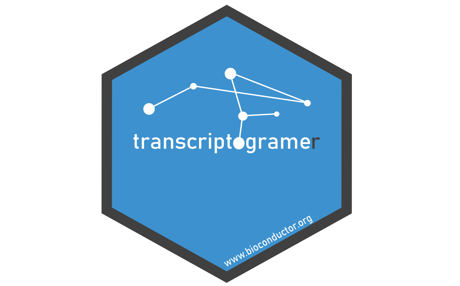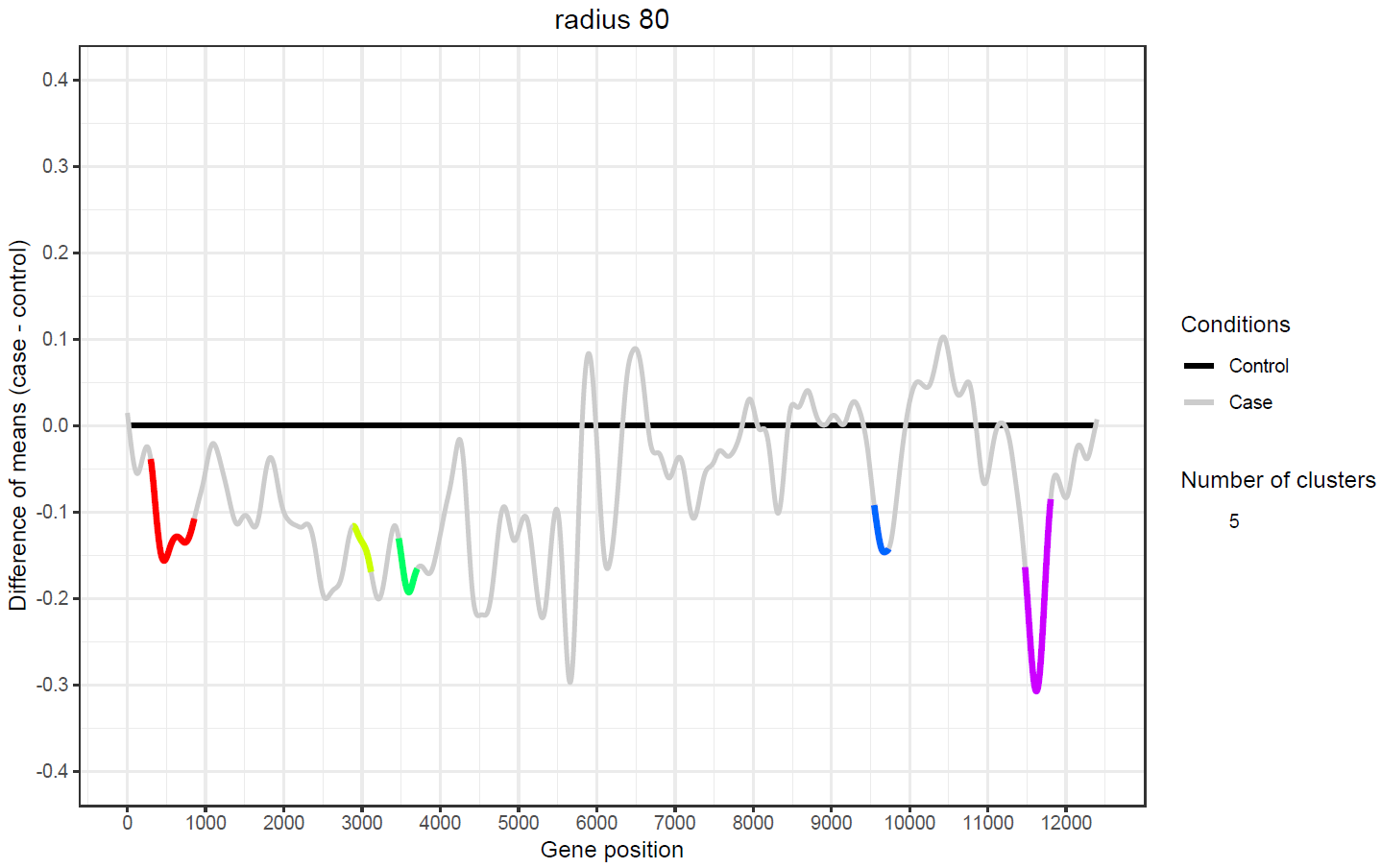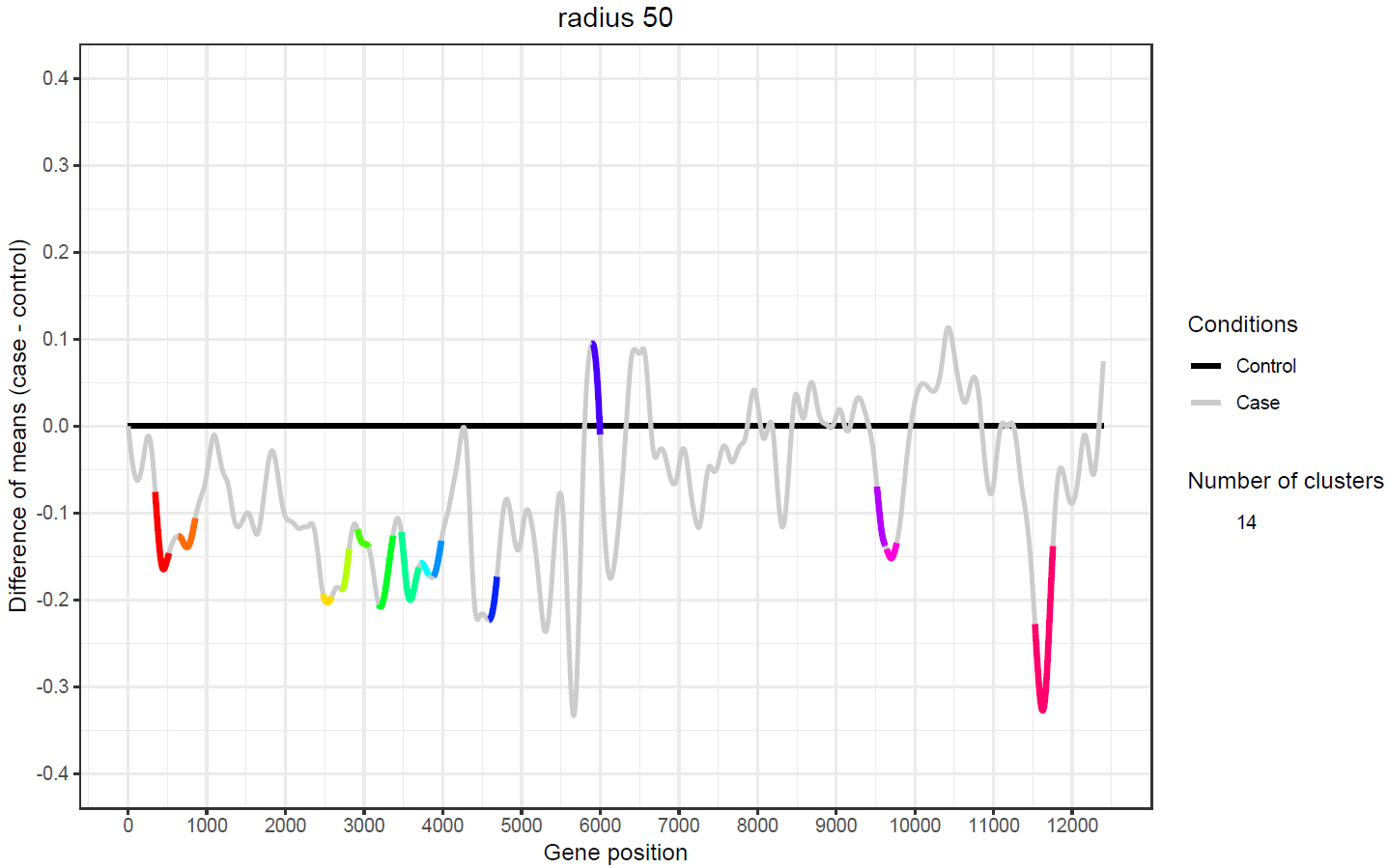# Contents

Version: 1.6.0# 1 Overview

The transcriptogramer package (Morais (2019)) is designed for transcriptional analysis based on transcriptograms, a method to analyze transcriptomes that projects expression values on a set of ordered proteins, arranged such that the probability that gene products participate in the same metabolic pathway exponentially decreases with the increase of the distance between two proteins of the ordering. Transcriptograms are, hence, genome wide gene expression profiles that provide a global view for the cellular metabolism, while indicating gene sets whose expression are altered (da Silva (2014); Rybarczyk-Filho (2011); de Almeida (2016); Ferrareze (2017); Xavier (2017)).

Methods are provided to analyze topological properties of an interactome, to generate transcriptograms, to detect and to display differentially expressed gene clusters, and to perform a Gene Ontology enrichment analysis on these clusters.

As a set of ordered proteins is required in order to run the methods, datasets are available for four species (Homo sapiens, Mus musculus, Saccharomyces cerevisiae and Rattus norvegicus). Each species has three datasets, originated from STRINGdb release 10.5 protein network data, with combined scores greater than or equal to 700, 800 and 900 (see Hs900, Hs800, Hs700, Mm900, Mm800, Mm700, Sc900, Sc800, Sc700, Rn900, Rn800 and Rn700 datasets). Custom sets of ordered proteins can be generated from protein network data using The transcriptogramer on Windows or Linux.

# 2 Quick start

The first step is to create a Transcriptogram object by running the `transcriptogramPreprocess()` method. This example uses a subset of the Homo sapiens protein network data, from STRINGdb release 10.5, containing only associations of proteins of combined score greater than or equal to 900 (see Hs900 and association datasets).

``````library(transcriptogramer)
t <- transcriptogramPreprocess(association = association, ordering = Hs900)``````

## 2.1 Topological analysis

There are two methods to perform topological analysis, `connectivityProperties()` calculates average graph properties as function of node connectivity, and `orderingProperties()` calculates graph properties projected on the ordered proteins. Some methods, such as orderingProperties(), uses a window, region of n (radius * 2 + 1) proteins centered at a protein, whose radius changes the output. The Transcriptogram object has a radius slot that can be setted during, or after, its preprocessing (see Transcriptogram-class documentation).

``````## during the preprocessing

## creating the object and setting the radius as 0
t <- transcriptogramPreprocess(association = association, ordering = Hs900)

## creating the object and setting the radius as 80
t <- transcriptogramPreprocess(association = association, ordering = Hs900,
``````## after the preprocessing

## modifying the radius of an existing Transcriptogram object

## getting the radius of an existing Transcriptogram object

As window related metrics are affected by the radius, the output of the orderingProperties() method changes depending on the content of the radius slot. A window modularity value close to 1 indicates dense connections between the genes inside the window, as well as sparse connections between these genes and the other genes in the network. Note that the sum of the window modularity increased using the radius 80.

``````oPropertiesR50 <- orderingProperties(object = t, nCores = 1)

## this output is partially different comparing to oPropertiesR50
oPropertiesR80 <- orderingProperties(object = t, nCores = 1)

sum(oPropertiesR50\$windowModularity)``````
`` 3346.246``
``sum(oPropertiesR80\$windowModularity)``
`` 4249.471``

However, the connectivityProperties() method does not use a window, thus, its output is not affected by the radius slot.

``cProperties <- connectivityProperties(object = t)``

## 2.2 Transcriptogram

A transcriptogram is generated in two steps and requires expression values, from microarray or RNA-Seq assays (log2-counts-per-million), and a dictionary. This example uses the datasets GSE9988, which contains normalized expression values of 3 cases and 3 controls (GSM252443, GSM252444, GSM252445, GSM252465, GSM252466 and GSM252467 respectively), and GPL570, a mapping between ENSEMBL Peptide ID and Affymetrix Human Genome U133 Plus 2.0 Array probe identifier.

The methods to generate a transcriptogram are `transcriptogramStep1()` and `transcriptogramStep2()`. The transcriptogramStep1() assigns to each protein, of each transcriptome sample, the average of the expression values of all the identifiers related to it.

``````t <- transcriptogramStep1(object = t, expression = GSE9988,
dictionary = GPL570, nCores = 1)
t2 <- t``````

To each position of the ordering, the transcriptogramStep2() method assigns a value equal to the average of the expression values inside a window, which considers periodic boundary conditions to deal with proteins near the ends of the ordering, in order to reduce random noise.

``t <- transcriptogramStep2(object = t, nCores = 1)``

The Transcriptogram object has slots to store the outputs of the transcriptogramStep1() and transcriptogramStep2() methods, called transcriptogramS1 and transcriptogramS2 respectively. As the output of some methods are affected by the content of the transcriptogramS2 slot, it can be recalculated using the content of the transcriptogramS1 slot.

``````radius(object = t2) <- 50
t2 <- transcriptogramStep2(object = t2, nCores = 1)``````

## 2.3 Gene Ontology enrichment analysis

As nearby genes of a transcriptogram have a high probability to interact with each other, gene sets whose expression are altered can be identified using the limma package. The `differentiallyExpressed()` method uses the limma package to identify differentially expressed genes (the approaches voom and trend are supported for RNA-Seq), for the contrast “case-control”, grouping as a cluster a set of genes which positions are within a radius range specified by the content of the radius slot.

For this example, the p-value threshold for false discovery rate will be set as 0.01. If a species name is provided, the biomaRt package is used to translate the ENSEMBL Peptide ID to Symbol (Gene Name), alternatively, a data.frame with such content can be provided. The levels argument classify the columns of the transcriptogramS2 slot referring to samples, as there are 6 columns (see dataset GSE9988), is created a logical vector that uses TRUE to label the columns referring to controls samples, and FALSE to label the columns referring to case samples.

``````## trend = FALSE for microarray data or voom log2-counts-per-million
## the default value for trend is FALSE
levels <- c(rep(FALSE, 3), rep(TRUE, 3))
t <- differentiallyExpressed(object = t, levels = levels, pValue = 0.01,
trend = FALSE, title = "radius 80")``````
``````## the radius 50 will affect the output significantly
t2 <- differentiallyExpressed(object = t2, levels = levels, pValue = 0.01,
species = DEsymbols, title = "radius 50")``````
``````## using the species argument to translate ENSEMBL Peptide IDs to Symbols
## Internet connection is required for this command
t <- differentiallyExpressed(object = t, levels = levels, pValue = 0.01,
species = "Homo sapiens", title = "radius 80")

## translating ENSEMBL Peptide IDs to Symbols using the DEsymbols dataset
t <- differentiallyExpressed(object = t, levels = levels, pValue = 0.01,
species = DEsymbols, title = "radius 80")``````

This method also produces a plot referring to its output. Each cluster detected is represented by a color. The genes that are above the horizontal black line are upregulated, and the genes that are below are downregulated.The differentially expressed genes identified by this method are stored in the DE slot of the Transcriptogram object, its content can be obtained using the DE method. By default, the p-values are adjusted by the Benjamini-Hochberg procedure. Note that the differential expression on the object of radius 80 detected less clusters, but there are more windows centers significantly altered on it, thus, the clusters are more consistent. Therefore, the next methods will be performed only on the object of radius 80.

``````DE <- DE(object = t)
DE2 <- DE(object = t2)
nrow(DE)``````
`` 393``
``nrow(DE)/length(unique(DE\$ClusterNumber))``
`` 78.6``
``nrow(DE2)``
`` 421``
``nrow(DE2)/length(unique(DE2\$ClusterNumber))``
`` 30.07143``

The `clusterVisualization()` method uses the RedeR package to display graphs of the differentially expressed clusters and returns an object of the RedPort Class, allowing interactions through methods of the RedeR package. This method requires the Java Runtime Environment (>= 6).

``rdp <- clusterVisualization(object = t)``